# Water Demand Formula for Fire Fighting – Environmental Engineering

## Water Demand Formula for Fire Fighting – Environmental Engineering

### FIRE DEMAND FORMULA

Water required for fire fighting is usually known as fire demand . Rate of fire demand is treated as a function of population and is worked out on the basis of empirical formula as follow-

#### 1. Kuichling Formula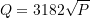where ,

Q = Amount of water required in liters/minute

P = Population in thousand

#### 2. Freeman Formula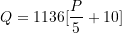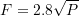where

Q = Amount of water required in liters/minute

P = Population in thousand

F = number of simultaneous fire streams

#### 3. National Board of Fire Under Writers Formula

##### (A) For Central Congested High Valued City

(1) Where Population < 200000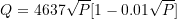(2) Where Population > 200000

 For First Fire(lit/minute)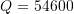For Second Fire(lit/minute) Q=9100 to 36400
##### (B) For Residential City
No. Type of Building Amount of Water Required (Q)
1. Small or Low Buildings Q=2200 lit/minute
2. Larger or higher buildings Q=4500 lit/minute
3. Apartment, High Value Residences,Tenements Q=7650 to 13500  lit/minute
4. Three Storeyed Buildings in Density Builtup Sections Q=27000 lit/minute

#### 4. Buston’s Formula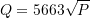where

Q = Amount of water required in liters/minute

P = Population in thousand

#### 5.  Frequency of fire may occur

The above formulae for water demand donot take into account the frequency of fire may occur.It may be determined from the following expression :-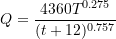where

t = Duration of fire in minutes

T =Period of occurance of fire in years

Note:- The recommended minimum values for the above formula are t=30minutes, T = 1 year

Updated: January 9, 2023 — 9:28 pm